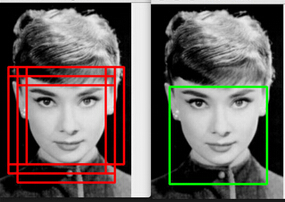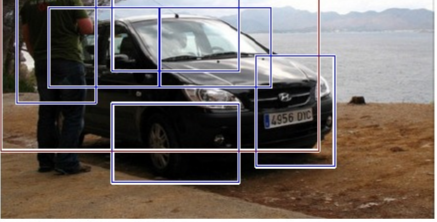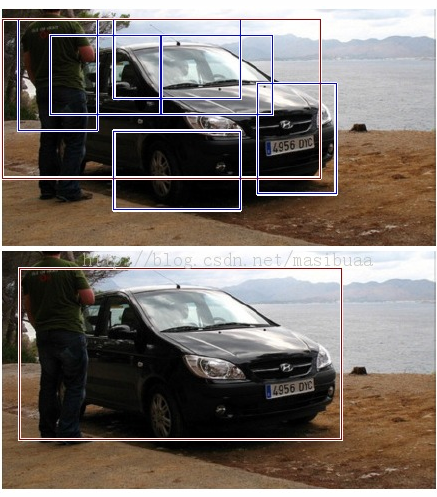# 非极大抑制（Non-maximum suppression）

## 一、Nms主要目的

在物体检测非极大抑制应用十分广泛，主要目的是为了消除多余的框，找到最佳的物体检测的位置。(1)从最大概率矩形框F开始，分别判断A~E与F的重叠度IOU是否大于某个设定的阈值;

(2)假设B、D与F的重叠度超过阈值，那么就扔掉B、D；并标记第一个矩形框F，是我们保留下来的。

(3)从剩下的矩形框A、C、E中，选择概率最大的E，然后判断E与A、C的重叠度，重叠度大于一定的阈值，那么就扔掉；并标记E是我们保留下来的第二个矩形框。## 二、原理

非极大抑制，顾名思义就是把非极大值过滤掉（抑制）。下面我就R-CNN或者SPP_net中的matlab源码来进行解释。

function picks = nms_multiclass(boxes, overlap)
%%boxes为一个m*n的矩阵，其中m为boundingbox的个数，n的前4列为每个boundingbox的坐标，格式为
%%（x1,y1,x2,y2）；第5:n列为每一类的置信度。overlap为设定值，0.3,0.5 .....
x1 = boxes(:,1);%所有boundingbox的x1坐标
y1 = boxes(:,2);%所有boundingbox的y1坐标
x2 = boxes(:,3);%所有boundingbox的x2坐标
y2 = boxes(:,4);%所有boundingbox的y2坐标

area = (x2-x1+1) .* (y2-y1+1); %每个%所有boundingbox的面积

picks = cell(size(boxes, 2)-4, 1);%为每一类预定义一个将要保留的cell
for iS = 5:size(boxes, 2)%每一类单独进行
s = boxes(:,iS);
[~, I] = sort(s);%置信度从低到高排序
pick = s*0;
counter = 1;
while ~isempty(I)
last = length(I);
i = I(last);
pick(counter) = i;%无条件保留每类得分最高的boundingbox
counter = counter + 1;

xx1 = max(x1(i), x1(I(1:last-1)));
yy1 = max(y1(i), y1(I(1:last-1)));
xx2 = min(x2(i), x2(I(1:last-1)));
yy2 = min(y2(i), y2(I(1:last-1)));

w = max(0.0, xx2-xx1+1);
h = max(0.0, yy2-yy1+1);

inter = w.*h;
o = inter ./ (area(i) + area(I(1:last-1)) - inter);%计算得分最高的那个boundingbox和其余的boundingbox的交集面积
I = I(o<=overlap);%保留交集小于一定阈值的boundingbox
end

pick = pick(1:(counter-1));
picks{iS-4} = pick;%保留每一类的boundingbox
end